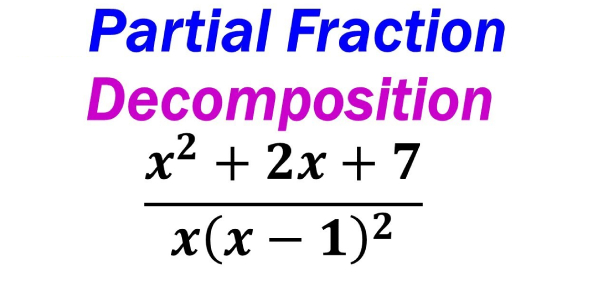# Math Trivia Quiz On Partial Fraction Decomposition

10 Questions | Total Attempts: 662SettingsHow much do you know about partial fraction decomposition? Mathematical equations of a rational function for solving problems where both numerator and denominator are polynomial equations, its importance lies in the fact that it provides an algorithm for computing anti-derivative of a rational function, take a quiz to learn more about partial fraction.

• 1.
A good way of solving a polynomial equation involving numerator and denominator is to apply partial fraction, what is partial fraction?
• A.

The decomposition process of starting with simplified answer

• B.

Taking answer back apart of decomposing

• C.

Decomposing the final expression into its initial polynomial fractions

• D.

All of the above

• 2.
Calculus question of Laplace transform or inverse Laplace to solve them depending on the problem given, what is the partial fraction in integration?
• A.

Partial fraction decomposition

• B.

Linear factors

• C.

Evaluation expressed in partial fraction

• D.

None of the above

• 3.
Laplace transform has always been applied to partial fraction question and its table can be used to solve equations using any of the formulae that applies to the problem, describe inverse Laplace transform?
• A.

If a function f(s) has inverse Laplace transform f(t)

• B.

Then, f(t) is uniquely determined

• C.

Considering functions that differs on a point set having Lebesgue measure zero as the same

• D.

All of the above

• 4.
In mathematics, there are rational numbers, irrational numbers, and rational functions, when is a function said to be a rational function?
• A.

Any function that can be defined by a rational fraction

• B.

An algebraic function

• C.

When both numerator and denominator are polynomials

• D.

All of the above

• 5.
Solving a partial fraction involves steps and in decomposition, you start with a proper rational expression, factor the bottom into linear factors, then?
• A.

Write out a partial fraction for each factor

• B.

Multiply the whole equation by the bottom

• C.

Solve for the coefficient by substituting zeros of the bottom

• D.

All of the above

• 6.
Solving partial fraction always involves substitution down the line in the process of integration and differentiation, what is integration by substitution?
• A.

U-substitution for finding integrals

• B.

Use integration

• C.

Antiderivative

• D.

None of the above

• 7.
In the process of solving partial fraction, the need to decompose equation by parts arises for easy problem solving, what is integration by parts?
• A.

Used when two functions multiplied together

• B.

Antiderivative

• C.

U-substitution

• D.

All of the above

• 8.
The most basic form of substitution when an integral contains some function and derivative, that is, for an integral of the form, what substitution is this?
• A.

U-substitution

• B.

V-substitution

• C.

Substitution by parts

• D.

None of the above

• 9.
Several rules apply when solving a partial fraction and this can only be used when the equation can be taken apart using a certain mathematical rule, what is chain rule?
• A.

To get derivative of a function

• B.

For differentiating compositions of functions

• C.

Denotation of h(x)

• D.

None of the above

• 10.
How do you in integrate LN X? if: In(x) dx. set u = In(x), dv=dx, then we find. du = (1/x)dx, v=x.
• A.

Substitute In(x) dx=u dv.

• B.

Use integration by parts = uv – yv du

• C.

Substitute u=In(x), v=x, and du=(1/x)dx

• D.

All of the above

Related TopicsBack to top